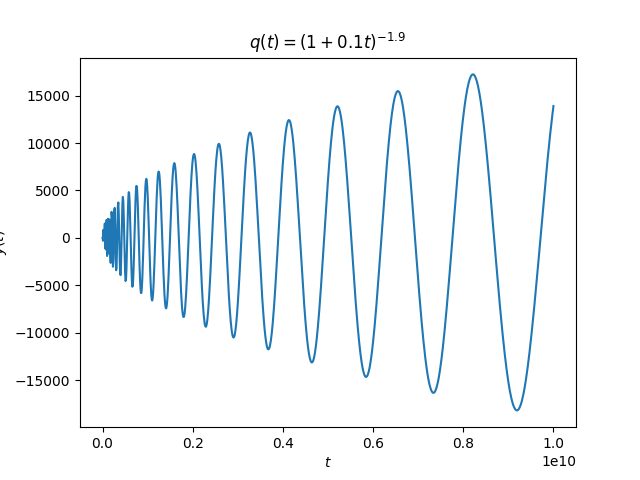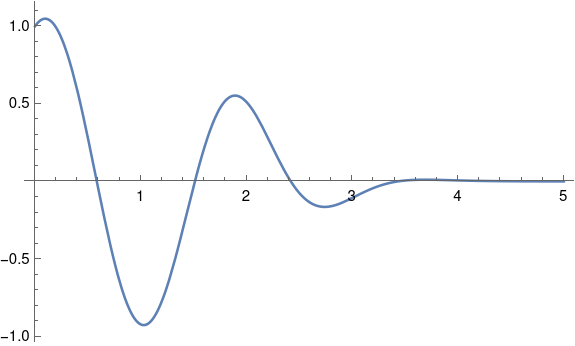# A closer look at zero spacing

A few days ago I wrote about Kneser’s theorem. This theorem tells whether the differential equation

u ″(x) + h(x) u(x) = 0

will oscillate indefinitely, i.e. whether it will have an infinite number of zeros.

Today’s post will look at another theorem that gives more specific information about the spacing of the zeros.

Kneser’s theorem said that the growth rate of h determines the oscillatory behavior of the solution u. Specifically, if h grows faster than ¼x-2 then oscillations will continue, but otherwise they will eventually stop.

## Zero spacing

A special case of a theorem given in  says that an upper bound on h gives a lower bound on zero spacing, and a lower bound on h gives an upper bound on zero spacing.

Specifically, if h is bound above by M, then the spacing between zeros is no more than π/√M. And if h is bound below by M′, then the spacing between zeros is no less than π/√M′.

Let’s look back a plot from the post on Kneser’s theorem:The spacing between the oscillations appears to be increasing linearly. We could have predicted that from the theorem in this post. The coefficient of the linear term is roughly on the order of 1/x² and so the spacing between the zeros is going to be on the order of x.

Specifically, in the earlier post we looked at

h(x) = (1 + x/10)p

where p was 1.9 and 2.1, two exponents chosen to be close to either side of the boundary in Kneser’s theorem. That post used q and t rather than h and x, but I changed notation here to match .

Suppose we’ve just seen a zero at x*. Then from that point on h is bounded above by h(x*) and so the distance to the next zero is bounded below by π/√h(x*). That tells you where to start looking for the next zero.

Our function h is bounded below only by 0, and so we can’t apply the theorem above globally. But we could look some finite distance ahead, and thus get a positive lower bound, and that would lead to an upper bound on the location of the next zero. So we could use theory to find an interval to search for our next zero.

## More general theorem

The form of the theorem I quoted from  was a simplification. The full theorem considers the differential equation

u ″(x) + g(xu′(x) +  h(x) u(x) = 0

The more general theorem looks at upper and lower bounds on

h(x) – ½ g′(x) – ¼ g(x)².

but in our case g = 0 and so the hypotheses reduced to bounds on just h.

## Example

Exercise 3 from  says to look at the zeros of solution to

u ″(x) + ½x²  u ′ + (10 + 2x) u(x) = 0.

Here we have

h(x) – ½ g ′(x)- ¼ g(x)² = 10 + 2xx – 1  = 9 + x

and so the lower bound of 9 tells us zeros are spaced at most π/3 apart.

Let’s look at a plot of the solution using Mathematica.

```    s = NDSolve[{u''[x] + 0.5 x^2 u'[x] + (10 + 2 x) u[x] == 0,
u == 1, u' == 1 }, u, {x, 0, 5}]
Plot[Evaluate[u[x] /. s], {x, 0, 5}]
```

This produces the following.There are clearly zeros between 0 and 1, between 1 and 2, and between 2 and 3. It’s hard to see whether there are any more. Let’s see exactly where the roots are that we’re sure of.

```    FindRoot[u[x] /. s, {x, 0, 1}]
{x -> 0.584153}

FindRoot[u[x] /. s, {x, 1, 2}]
{x -> 1.51114}

FindRoot[u[x] /. s, {x, 2, 3}]
{x -> 2.41892}
```

The distance between the first two zeros is 0.926983 and the distance between the second and third zeros is 0.90778. This is consistent with our theorem because π/3 > 1 and the spacing between our zeros is less than 1.

Are there more zeros we can’t see in the plot above? When I asked Mathematica to evaluate

`    FindRoot[u[x] /. s, {x, 2, 4}]`

it failed with several warning messages. But we know from our theorem that if there is another zero, it has to be less than a distance of π/3 from the last one we found. We can use this information to narrow down where we want Mathematica look.

`    FindRoot[u[x] /. s, {x, 2.41892, 2.41892 + Pi/3}]`

Now Mathematica says there is indeed another solution.

`    {x -> 3.42523}`

Is there yet another solution? If so, it can’t be more than a distance π/3 from the one we just found.

`    FindRoot[u[x] /. s, {x, 3.42523, 3.42523 + Pi/3}]`

This returns 3.42523 again, so Mathematica didn’t find another zero. Assuming Mathematica is correct, this means there cannot be any more zeros.

On the interval [0, 5] the quantity in the theorem is bound above by 14, so an additional zero would need to be at least π/√14 away from the latest one. So if there’s another zero, it’s in the interval [4.26486, 4.47243]. If we ask Mathematica to find a zero in that interval, it fails with warning messages. We can plot the solution over that interval and see that it’s never zero.

 Protter and Weinberger. Maximum Principles in Differential Equations. Springer-Verlag. 1984. Page 46.